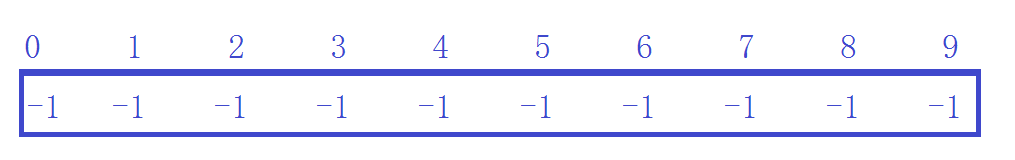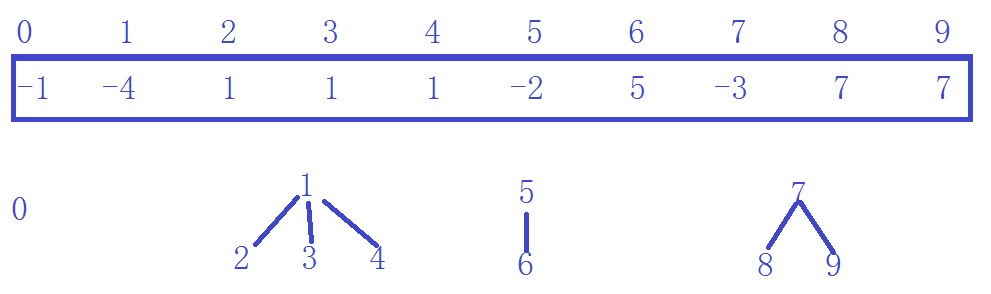# 并查集class UnionFindSet//并查集
{
public:
UnionFindSet(size_t n)
{
_set.resize(n, -1);
}
int FindRoot(int x)
{
while (_set[x] >= 0)
x = _set[x];
return x;
}
void Union(int x1, int x2)
{
int root1 = FindRoot(x1);
int root2 = FindRoot(x2);
if (root1 != root2)
{
_set[root1] += _set[root2];
_set[root2] = root1;
}
}
bool IsIn(int x1, int x2)
{
int root1 = FindRoot(x1);
int root2 = FindRoot(x2);
if (root1 == root2)
return true;
return false;
}
protected:
vector<int> _set;
};
07-296411
06-09736009-291474
06-1221万+
08-093803
08-055528
07-206287